Signed Number Arithmetic

Signed Number Arithmetic

In math we first learn that the symbol + tells us to add while the symbol - tells us to subtract.

When these signs are attached to numbers, however, they show us whether the value of that number is greater than 0 (+1) or less than 0 (-1). For example, when you see 4 or +4 (a.k.a. "positive four"), that number is 4 points greater than 0 on the number line below. On the other hand, a minus sign in front of a 4 makes it -4 (a.k.a. "negative four"). That number has a value that is 4 points less than 0 on the number line below.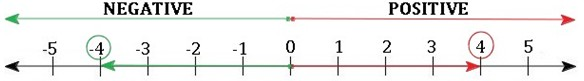These values of "+4" (positive four) and " - 4" (negative four) are called signed numbers.

Operations with Signed Numbers

When you are adding two or more numbers with the same sign, their sum will also have that sign.

(+23) (+12) = (+35)

(-157) + (-13) = (-170)

(+45.2) (+0.8) (+4.5) = (+50.5)

When you are adding two numbers with different signs, find the difference between those numbers by subtracting the smaller number from the larger number. The resultant value will then have the sign of the larger number

(+8) (-12) = (-4)

(-3) (+5) = (+2)

(-3.50) (+3.51) = (+0.01)

We can use the number line to show the sum of signed numbers.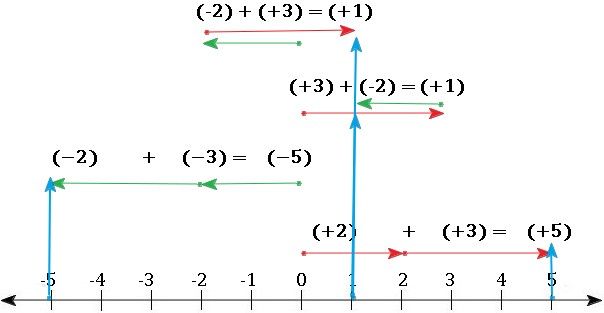Subtraction

When you subtract two numbers with the same sign, change the sign of the second number and add both numbers together

(+23) - (+12) = (+23) (-12) = (+11)

(-157) - (-13) = (-157) (+13) = (-144)

(+45.2) - (+4.5) = (+45.2) (-4.5) = (+40.7)

The same should be done when you subtract two numbers with a different sign. In this case after you change the sign of the second number, the resultant value will have the sign of the larger number.

(+8) - (-12) = (+8) (+12) = (+20)

(-3) - (+5) = (-3) (-5) = (-8)

(-3.50) - (+3.51) = (-3.50) + (-3.51) = (+7.01)

Multiplication

When you multiply two numbers with the same sign, the product will be positive.

(+23)  (+12) = (+276)

(-157)  (-13) = (+2041)

(+45.2)  (+4.5) = (+203.4)

When you multiply two numbers with different signs, the product will be negative.

(+8)  (-12) = (-96)

(-3)  (+5) = (-15)

(-3.50)  (+3.51) = (-12.285)

What about three or more numbers?

When you have three or more numbers, you can first find the product of all of the factors.

You can then count the number of negative factors in the problem: if there is an "even" number of negative numbers, then the product will be positive.

(+2)  (-2)  (-2) = (+8)

If there's an "odd" number of negative numbers, then the resultant will be negative.

(+2)  (+2)  (-2) = (-8)

Division

For division the rules for signs are the same as the rules for multiplication. When you divide two numbers with the same signs, the quotient will be positive.

(+24)  (+12) = (+2)

(-156)  (-13) = (+12)

(+45.2)  (+4.5) =

When you divide two numbers with different signs, the quotient will be negative.

(+9)(-12) = (-0.75)

(-3)  (+5) = (-0.6)

(-3.50) (+3.51) =

Finally, the same rule applies when dividing 3 or more numbers. If we have an "even" number of negative numbers, then the quotient will be positive.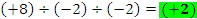If we have an "odd" number of negative numbers, then the quotient will be negative.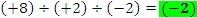Add and subtract the following numbers: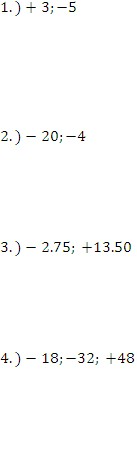Multiply and divide the following values.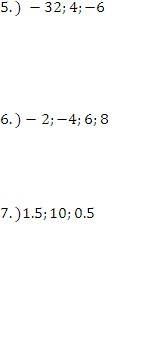1. -2 and 8

2. -24 and -16

3. 10.75 and -16.25

4. -2 and -34

5. 768 and 8/6 or 4/3

6. 384 and 1/96

7. 7.5 and 3/10